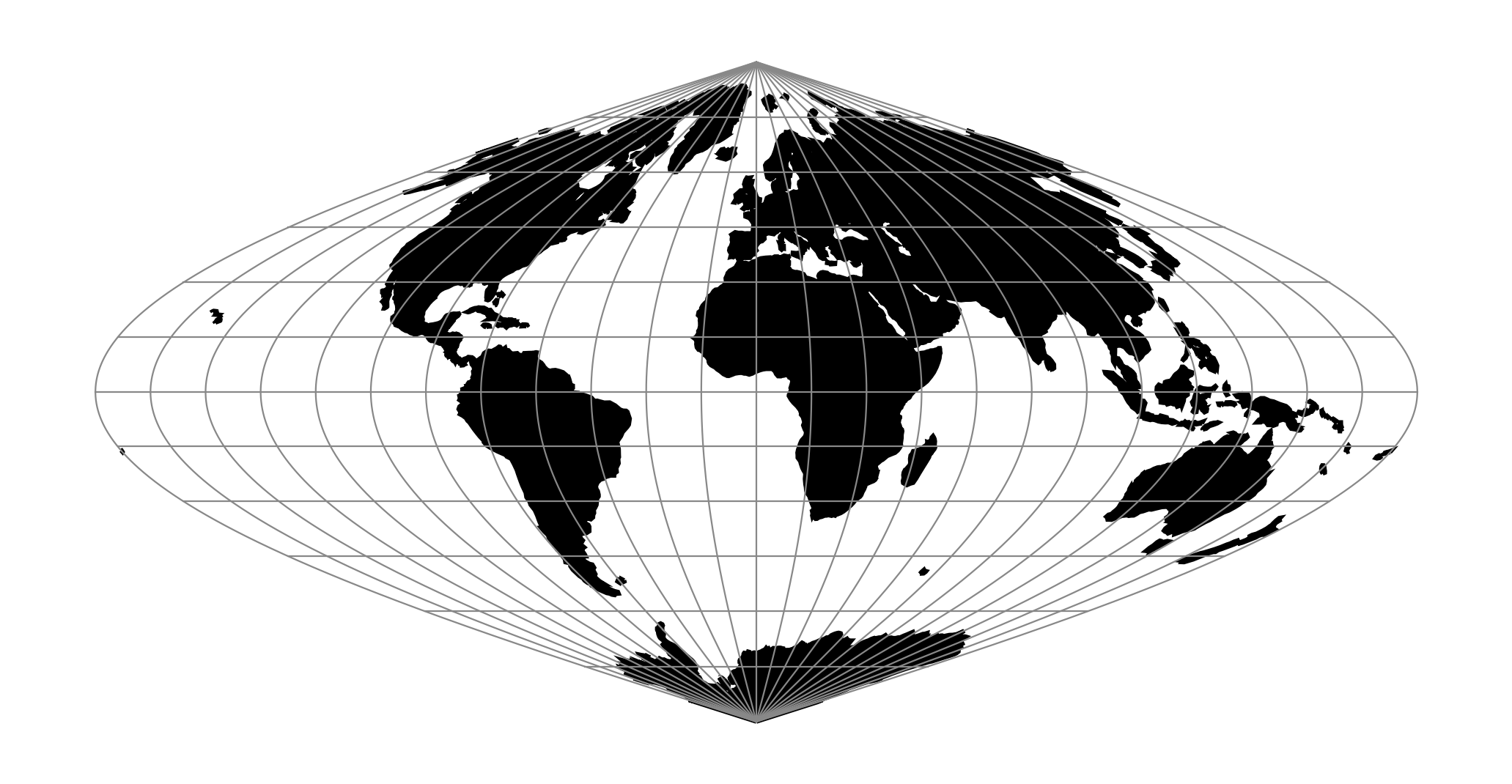# Sinusoidal (Sanson-Flamsteed)¶proj-string: +proj=sinu

MacBryde and Thomas developed generalized formulas for several of the pseudocylindricals with sinusoidal meridians:

$x = C\lambda(m+cos\theta) / ( m + 1)$
$y = C\theta$
$C = \sqrt { (m + 1 ) / n }$

## Parameters¶

Note

All parameters are optional for the Sinusoidal projection.

+lon_0=<value>

Longitude of projection center.

Defaults to 0.0.

+R=<value>

Radius of the sphere given in meters. If used in conjunction with +ellps +R takes precedence.

+x_0=<value>

False easting.

Defaults to 0.0.

+y_0=<value>

False northing.

Defaults to 0.0.